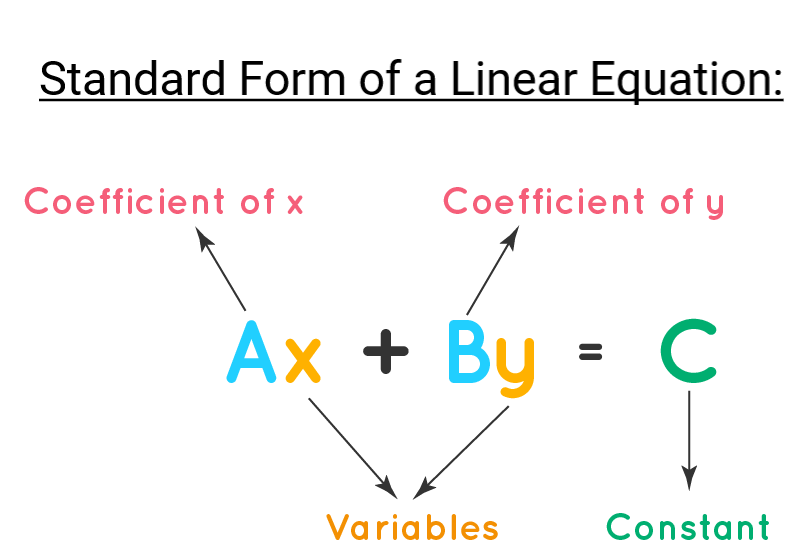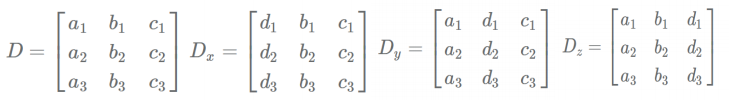Courses

# Linear Equations - Important Formulas, Quantitative Aptitude GMAT Notes | EduRev

## Banking Exams : Linear Equations - Important Formulas, Quantitative Aptitude GMAT Notes | EduRev

The document Linear Equations - Important Formulas, Quantitative Aptitude GMAT Notes | EduRev is a part of the Banking Exams Course Quantitative Aptitude for GMAT.
All you need of Banking Exams at this link: Banking Exams

Introduction

• Linear equations is one of the foundation topics in the Quant section of CAT.
• Hence, the fundamentals of this concept are useful in solving the questions of the other topics by assuming the unknown values as variables.
• Be careful of silly mistakes in this topic as that is how students generally lose marks here.
• Generally, the number of equations needed to solve the given problem is equal to the number of variables.

What is a Linear Equation?A linear equation is an equation which gives straight line when plotted on

a graph.

• Linear equations can be of one variable or two variable or three variable.
• Let a, b, c and d are constants and x, y and z are variables. A general form of single variable linear equation is ax+b = 0.
• A general form of two variable linear equation is ax+by = c.
• A general form of three variable linear equation is ax+by+cz = d.

➢ Equations with Two Variables

• Consider two equations ax+by = c and mx+ny = p. Each of these equations represent two lines on the x-y co-ordinate plane. The solution of these equations is the point of intersection.
• If a / m = b / n ≠ c / p then the slope of the two equations is equal and so they are parallel to each other. Hence, no point of intersection occurs. Therefore no solution.
• If a / m ≠ b / n then the slope is different and so they intersect each other at a single point. Hence, it has a single solution.
• If a / m = b / n = c / p then the two lines are same and they have infinite points common to each other. So, infinite solutions occurs.

Try yourself:The pair of equations 3x – 5y = 7 and – 6x + 10y = 7 have:

➢ General Procedure to Solve Linear Equations

• Aggregate the constant terms and variable terms.
• For equations with more than one variable, eliminate variables by substituting equations in their place.
• Hence, for two equations with two variables x and y, express y in terms of x and substitute this in the other equation.
• Example: Let x + y = 14 and x + 4y = 26 then x = 14-y (from equation 1) substituting this in equation 2, we get 14 - y + 4y = 26. Hence, y = 4 and x = 10.
• For equations of the form ax+by = c and mx+ny = p, find the LCM of b and n. Multiply each equation with a constant to make the y term coefficient equal to the LCM. Then subtract equation 2 from equation 1.
• Example: Let 2x+3y = 13 and 3x+4y = 18 are the given equations (1) and (2).
⇒ LCM of 3 and 4 is 12.
⇒ Multiplying (1) by 4 and (2) by 3, we get 8x+12y = 52 and 9x+12y = 54.
⇒ (2) - (1) gives x = 2, y = 3
• If the system of equations has n variables with n-1 equations then the solution is indeterminate.
• If system of equations has n variables with n-1 equations with some additional conditions like the variables are integers then the solution may be determinate.
• If system of equations has n variables with n-1 equations then some combination of variables may be determinable.
• Example: If ax+by+cz = d and mx+ny+pz = q, if a, b, c are in Arithmetic progression and m, n and p are in AP then the sum x+y+z is determinable.

➢ Equations with Three Variables

• Let the equations be a1x + b1y + c1z = d1, a2x+b2y+c2z = d2 and a3x+b3y+c3z = d3 . Here we define the following matrices:• If Determinant of D ≠ 0, then the equations have a unique solution.
• If Determinant of D = 0, and at least one but not all of the determinants Dx , Dy or Dz is zero, then no solution exists.
• If Determinant of D = 0, and all the three of the determinants Dx , Dy and Dz are zero, then there are infinitely many solution exists.
• Determinant can be calculated by D = a1(b2c3 -c2b3) - b1(a2c3 - c2a3) + c1(a2b3 -b2a3)
Offer running on EduRev: Apply code STAYHOME200 to get INR 200 off on our premium plan EduRev Infinity!

## Quantitative Aptitude for GMAT

80 videos|99 docs|175 tests

,

,

,

,

,

,

,

,

,

,

,

,

,

,

,

,

,

,

,

,

,

,

,

,

;